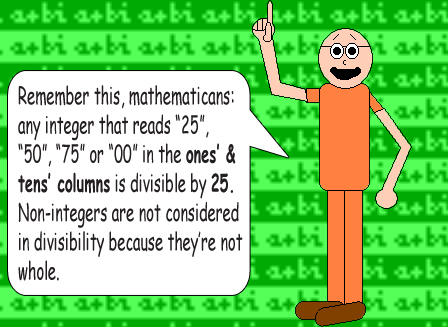# A Shortcut for Multiplying by 25

## Here's a quick way to multiply numbers by 25: First, divide the multiplicand by 4, and then multiply that quotient by 100.

Note: You can multiply by 100 first, and then divide by 4 to get the same result since multiplication & division are on the same calculation level!

## Examples:

25 × 17 = 425

because

17 ÷ 4 = 4.25; 4.25 × 100 = 425

25 × 60 = 1,500

because

60 ÷ 4 = 15; 15 × 100 = 1,50025 × 153 = 3,825

because

153 ÷ 4 = 38.25; 38.25 × 100 = 3,825

This trick works since 25 = 100 ÷ 4. Frankly, it still works even if the multiplicand is not an integer, but these examples are here to teach you how to multiply integers by the number 25!